# CLASS 10 MATH NCERT SOLUTIONS FOR CHAPTER – 6 TRIANGLES EX – 6.6

## Triangles

Question 1.
In the given figure, PS is the bisector of ∠QPR of ∆PQR. Prove that

Solution:Question 2.
In the given figure, D is a point on hypotenuse AC of ∆ABC, DM ⊥ BC, and DN ⊥ AB. Prove that:

(i) DM2 = DN X MC
(ii) DN2 = DM X AN

Solution:

Question 3.
In the given figure, ABc is triangle in which ∠ABC > 90° and AD ⊥ CB produced. Prove that AC2 = AB2 + BC2  + 2BC X BD

Solution:

Question 4.
In the given figure, ABC is a triangle in which ∠ABC 90° and AD ⊥ CB. Prove that AC2 = AB2 + BC2 – 2BC X BD

Solution:

Question 5.
In the given figure, Ad is a median of a triangle ABC and AM ⊥ BC. Prove that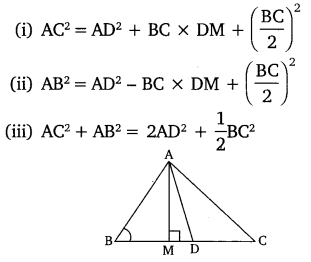Solution: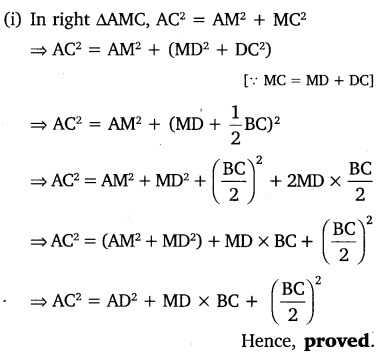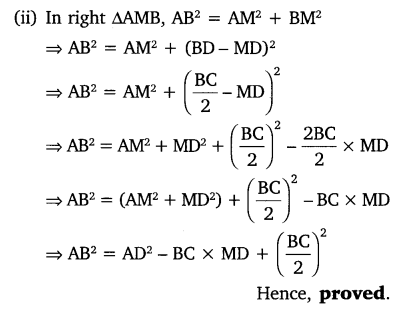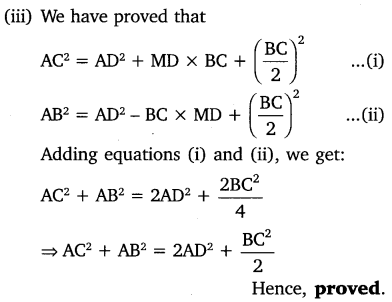Question 6.
Prove that the sum of the squares of the diagonals of a parallelogram is equal to the sum of the squares of its sides.Solution:

Solutions :-

Question 7.
In the given figure, two chords AB and CD intersect each other at the point P. Prove that:

(i) ∆APC ~∆DPB
(ii)
AP X PB = CP X DP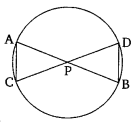Solution:

Question 8.
In the given figure, two chords Ab and CD of a circle intersect each other at the point P (when produced) outside the circle. Prove that:

(i) ∆PAC ~ ∆PDB
(ii)
PA X PB = PC X PD

Solution:

Question 9.
In the given figure, D is a point on side BC of ∆ABC, such that  Prove that AD is the bisector of ∆BAC.

Solution:

Question 10.
Nazima is fly fishing in a stream. The trip of her fishing rod is 1.8m above the surface of the water and the fly at the end of the string rests on the water 3.6m away and 2.4 m from a point directly under the trip of the rod. Assuming that her string (from the trip of the rod to the fly) is that, how much string does she have out (see the figure)? If the pills in the string at the rate of 5 cm per second, what will be the horizontal distance of the fly from her after 12 seconds?

Solution:

#### CLASS 10 MATH NCERT SOLUTIONS FOR CHAPTER – 7 Coordinate Geometry Ex – 7.1

Lorem ipsum dolor sit amet, consectetur adipiscing elit. Phasellus cursus rutrum est nec suscipit. Ut et ultrices nisi. Vivamus id nisl ligula. Nulla sed iaculis ipsum.

Company Name